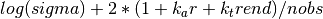# statsmodels.tsa.ar_model.ARResults¶

class statsmodels.tsa.ar_model.ARResults(model, params, normalized_cov_params=None, scale=1.0)[source]

Class to hold results from fitting an AR model.

Parameters: model : AR Model instance Reference to the model that is fit. params : array The fitted parameters from the AR Model. normalized_cov_params : array inv(dot(X.T,X)) where X is the lagged values. scale : float, optional An estimate of the scale of the model. Attributes aic : float Akaike Information Criterion using Lutkephol’s definition.bic : float Bayes Information Criterionbse : array The standard errors of the estimated parameters. If method is ‘cmle’, then the standard errors that are returned are the OLS standard errors of the coefficients. If the method is ‘mle’ then they are computed using the numerical Hessian. fittedvalues : array The in-sample predicted values of the fitted AR model. The k_ar initial values are computed via the Kalman Filter if the model is fit by mle. fpe : float Final prediction error using Lutkepohl’s definition ((n_totobs+k_trend)/(n_totobs-k_ar-k_trend))*sigma hqic : float Hannan-Quinn Information Criterion. k_ar : float Lag length. Sometimes used as p in the docs. k_trend : float The number of trend terms included. ‘nc’=0, ‘c’=1. llf : float The loglikelihood of the model evaluated at params. See AR.loglike model : AR model instance A reference to the fitted AR model. nobs : float The number of available observations nobs - k_ar n_totobs : float The number of total observations in endog. Sometimes n in the docs. params : array The fitted parameters of the model. pvalues : array The p values associated with the standard errors. resid : array The residuals of the model. If the model is fit by ‘mle’ then the pre-sample residuals are calculated using fittedvalues from the Kalman Filter. roots : array The roots of the AR process are the solution to (1 - arparams*z - arparams*z**2 -...- arparams[p-1]*z**k_ar) = 0 Stability requires that the roots in modulus lie outside the unit circle. scale : float Same as sigma2 sigma2 : float The variance of the innovations (residuals). trendorder : int The polynomial order of the trend. ‘nc’ = None, ‘c’ or ‘t’ = 0, ‘ct’ = 1, etc. tvalues : array The t-values associated with params.

Methods

 aic() bic() bse() fittedvalues() fpe() hqic() predict([start, end, dynamic]) Returns in-sample and out-of-sample prediction. pvalues() resid() roots() scale() sigma2()

Attributes

 extra_doc preddoc use_t